• 2019-01-10 15:57:13

//1、得到最小值
private static int min(int... is) {
int min = Integer.MAX_VALUE;
for (int i : is) {
if (min > i) {
min = i;
}
}
return min;
}

// 2、得到最小值
private static int min(int one, int two, int three) {
return (one = one < two ? one : two) < three ? one : three;
}java
更多相关内容
• 该实验使用模拟退火算法求取函数的最小值，matlab自编程实现。该实验可以观察到搜索点的过程，也可以自行修改参数。
• 资源包含以下内容： Sheffield的遗传算法工具箱 利用遗传算法求函数最小值.m
• 求下列二元函数的最大值，f(x1,x2)=x1^2+x2^2,x1与x2的取值区间为{0,1,2，...，7}
• MINMAX 查找第 k 个最小值或最大值及其索引。 用法： vals = minmax(data) % 找到最小值vals = minmax(data,k) % 找到第 k 个最小值vals = minmax(data,k,flag) % 找到第 k 个最大值[vals,loci] = minmax(:) [vals,...matlab
• 主要介绍了C#获取数组中最大最小值的方法,本文直接给出实例代码,需要的朋友可以参考下
• 今天小编就为大家分享一篇使用遗传算法求二元函数的最小值，具有很好的参考价值，希望对大家有所帮助。一起跟随小编过来看看吧
• 今天小编就为大家分享一篇python实现列表中最大最小值输出的示例，具有很好的参考价值，希望对大家有所帮助。一起跟随小编过来看看吧
• 主要介绍了JAVA得到数组中最大值和最小值的简单实例，需要的朋友可以参考下
• 主要介绍了linux shell实现求一个多维数组中的最大和最小值,需要的朋友可以参考下
• 主要介绍了java 求解二维数组列最小值的相关资料,需要的朋友可以参考下
• 1. 基于matlab实现的返回矩阵的最大值最小值及其对应的小标 2. 函数特别简单，直接输入矩阵或者向量，返回矩阵的最大值最小值及其下标
• ## 遗传算法求解函数最小值问题

万次阅读 多人点赞 2019-05-17 10:21:51
遗传算法求解函数最小值问题 继上一次用遗传算法求解TSP问题问题以后，万万没有想到，实验的时候，老师居然改了题目，改成了求解函数的最小值问题（有点坑哈），而且要求结果尽量的稳定，可以确定得到最小值，并且，...

# 遗传算法求解函数最小值问题

继上一次用遗传算法求解TSP问题问题以后，万万没有想到，实验的时候，老师居然改了题目，改成了求解函数的最小值问题（有点坑哈），而且要求结果尽量的稳定，可以确定得到最小值，并且，精度尽可能的高……虽然有点坑，不过老师还是简单的说明了一下基本的思路，思路和上一次没有太大的变化，唯一的难点就是怎样尽可能的提高解的精度。
不管怎样，终究在实验课的时候解决了这个问题，唯一的问题就是进度最多10的负六次方，老师说他的精度可以达到10的负几百次方（膜拜……）。
思路不变，选择用轮盘赌，变异要稍微变一下，具体会在下面讲解，废话少说，进入正题。.

## 问题描述：

设计高效遗传算法，求解下列函数在-5<=x1,x2<=5上的最小值：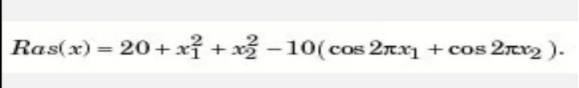在正式的开始求解之前，老师先将函数的图片展示了出来，如下图所示：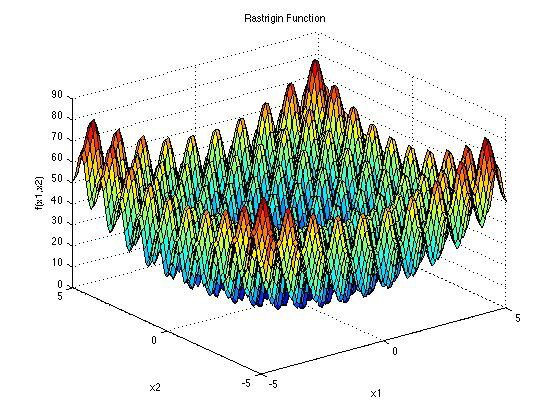并且公布结果，在（0,0）的位置取得最小值0。
在这个结果的基础上，我们开始试验。

## 代码编写：

首先是个体的类代码：

class problem1_individual(object):
def __init__(self, n1, n2):
self.gene = [n1, n2]
self.score = 0
pass


接着轮盘赌选择的代码，和上一次没有多少变化，同样是采用倒数的方式来作为个体的适配值，因为事先知道结果的最小值为0，所以采用倒数的方式很好，因为越接近0，倒数越大，被选中的概率就越大。
代码如下所示：

def roulette_wheel(self):
"""
轮盘赌普通方法得到被选中的基因型
:param individuals: 种群中各个基因类型的数量
:return: 返回被选中的基因型的代号
"""
all_individual = 0
for i in range(len(self.list)):
all_individual += 1 / self.list[i].score
probabilities = []
for i in range(len(self.list)):
probabilities.append((1 / self.list[i].score) / all_individual)
selected_individual = random.uniform(0, 1)
now_individual = 0.0
for ind, val in enumerate(probabilities):
now_individual += val
if now_individual > selected_individual:
return self.list[ind]


接着是交叉的代码。在编写之前还没有办法，个体是两个自变量，但是交叉怎么办？上网查了一下，有了思路，很简单，就是用不同的比例进行划分。
随机生成一0-1的数字，作为其中一个父个体的基因比例，再用1减去这个数字，得到另一个父个体的基因比例，两者相加得到的就是最后的孩子的基因序列：

def crossgene(self, parent1, parent2, index1, index2):
child = [0, 0]
child = parent1.gene * index1 + parent2.gene * index2
child = parent1.gene * index1 + parent2.gene * index2
return child

def crossover(self, father, mother):
"""
:param father: 需要进行遗传的父类个体
:param mother: 需要进行遗传的母类个体
:return:
"""
x = random.randint(0, 9999)
# 变异有概率，大于某个值就不发生，小于某个值就发生变异
k = 10000 * self.variationrate
if x < k:
# 进行单点交换
index1 = random.uniform(0, 1)
index2 = 1 - index1
child1 = self.crossgene(father, mother, index1, index2)
child2 = self.crossgene(mother, father, index1, index2)
else:
child1 = father.gene
child2 = mother.gene
return child1, child2


## 结果测试：

代码编写完毕，现在进行测试，看看结果如何：

from problem1_GA import problem1_GA

test = problem1_GA(1, 1)
test.initpopulation()
for i in range(10000):
test.next_generation()
print()
pass


迭代次数就采用10000次，看看最后的结果达到什么样的精度：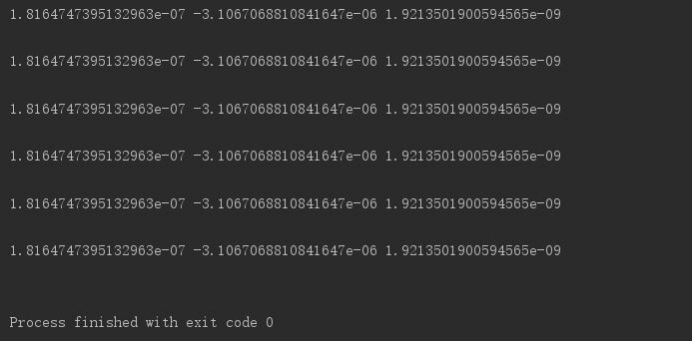运气不错，最后的结果精度可以达到10^-9次方的水平。当然这次是运气好，但是不是每一次都可以重现这种操作，我还会继续的进行代码的优化，下一次让结果有更好的精度。
当然这都是后话。
最后完整的代码就放在以下链接中
遗传算法求解函数最小值问题
提取码：v2y6
还有上一次的文章缺少的CSDN链接，是tomcat的安装包和eclipse插件：
CSDN—tomcat
谢谢大家阅读！

展开全文遗传算法
• 基于谷底最小值的阈值利用matlab平台实现所用图为基础雷纳图
• 该代码实现了运用差分进化算法解决目标函数的最小值，这里解决的是目标函数y=x*sin(10*PI*x)+2的最小值，读者可以根据自己的需要进行修改目标函数求解最小值，同时可以修改代码求解最大值。
• 本文件使用粒子群算法计算出二元函数的最小值，运行速度快。得到的最小值非常接近最优值。只需修改对应的函数表达式，根据所需要的区间，即可运行
• 在向量中寻找局部最小值或最大值的函数集，在向量中找到最接近某个值的点，以及清除所有内容的小函数。matlab
• 本文给大家汇总介绍的是获取JavaScript 数组中最大最小值的方法和示例，非常的详细和全面，希望对大家学习JavaScript能够有所帮助
• 在python中利用numpy创建一个array， 然后我们想获取array的最大值，最小值。可以使用一下方法： 一、创建数组 这样就可以获得一个array的最大值和最小值了。 并且可以利用np.where(np.max(a))来获得最大值，最小值...
• 今天小编就为大家分享一篇python寻找list中最大值、最小值并返回其所在位置的方法，具有很好的参考价值，希望对大家有所帮助。一起跟随小编过来看看吧
• 最小值． 函数图像如图（及程序）所示： clear all; close all; clc; x=0:0.01:10; y=x+10*cos(5*x)+7*sin(4*x); figure plot(x,y) grid on xlabel('x') ylabel('f(x)') title('f(x)=x+10*cos(5*x)+7*sin(4*x)')...

用标准遗传算法求函数：

的最小值．

函数图像如图（及程序）所示：

clear all;
close all;
clc;
x=0:0.01:10;
y=x+10*cos(5*x)+7*sin(4*x);
figure
plot(x,y)
grid on
xlabel('x')
ylabel('f(x)')
title('f(x)=x+10*cos(5*x)+7*sin(4*x)')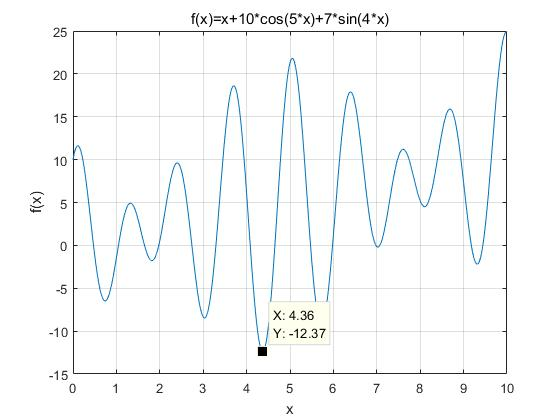仿真过程如下：

（１）初始化种群数目ＮＰ＝５０

染色体二进制编码长度Ｌ＝２０

最大进化代数Ｇ＝１００

交叉概率Ｐｃ＝０．８

变异概率ｐｍ＝０．０５

（２）ａ．产生初始化种群，将二进制编码转化为十进制；

ｂ．计算个体适应度，并且进行归一化操作；

ｃ．在选择操作中，选择基于轮盘赌的方式进行；

ｄ．在交叉和变异过程，选择基于概率的方式进行；

ｅ．产生新的种群，并把历代的最优个体留在新的种群之中；

ｆ．重复ｂ——ｅ过程，进行下一步遗传操作。

（３）遗产算法的终止条件判断：

Ｉ．判断是否满足终止条件，若满足，则终止搜索过程，输出最优值；若不满足则继续进行迭代；

ＩＩ．为了防止遗传过程过长，可以设置遗传代数控制搜索过程。

程序如下：

function result=func(x)
fit=x+10*cos(5*x)+7*sin(4*x);
result=fit;

%%%%%%%%%%%%%%求最小值%%%%%%%%%%%%%%%%%%%
%%%%%%%%%%参数初始化%%%%%%%%%%%%%%%%%%%%%%
clear all;%清变量
close all;%清图
clc;%清屏

NP=50;%种群规模为50个
L=20 ;%二进制字串长度
Pc=0.8;%交叉概率
Pm=0.05;%变异概率
G=100;%max generation
Xs=10;%上限
Xx=0;%下限
f=randint(NP,L);
%%%%%%%%%%%%%%%%%%%%%%%%%%%%%%%%%%%%%%%%%%%%%%%%%%%算法循环部分%%%%%%%%%%%%%
for k =1:G
for i=1:NP
U=f(i,:);
m=0;
for j=1:L
m=U(j)*2^(j-1)+m;
end
x(i)=Xx+m*(Xs-Xx)/(2^L-1);
Fit(i)=-func(x(i));
end
maxFit=max(Fit);%最大值
minFit=min(Fit);%最小值
rr=find(Fit==maxFit);
fBest=f(rr(1,1),:);%历代最小值
xBest=x(rr(1,1));
Fit=(Fit-minFit)/(maxFit-minFit);
%%%%基于轮盘赌的选择操作%%%%%%%
sum_Fit=sum(Fit);
fitvalue=Fit./sum_Fit;
fitvalue=cumsum(fitvalue);
ms=sort(rand(NP,1));
fiti=1;
newi=1;
while newi<=NP
if(ms(newi)<fitvalue(fiti))
nf(newi,:)=f(fiti,:);
newi=newi+1  ;
else
fiti=fiti+1;
end
end
%%%%基于概率的交叉操作%%%%%
for i=1:2:NP
p=rand;
if p<Pc
q=randint(1,L);
for j=1:L
if q(j)==1;
temp=nf(i+1,j);
nf(i+1,j)=nf(i,j);
nf(i,j)=temp;
end

end
end
end
%%%%基于概率的变异操作%%%

for m=1:NP
for n=1:L
r=rand(1,1);
if r<Pm
nf(m,n)=rand(1,1)*(Xs-Xx)+Xx;
end
end
end
f=nf;
f(1,:)=fBest;
trace(k)=-maxFit;

end
xBest;
figure
plot(trace)
xlabel('迭代次数')
ylabel('目标函数值')
title('适应度进化曲线')
x=xBest
y=-maxFit

运行结果：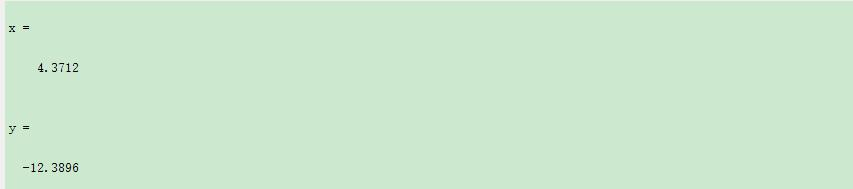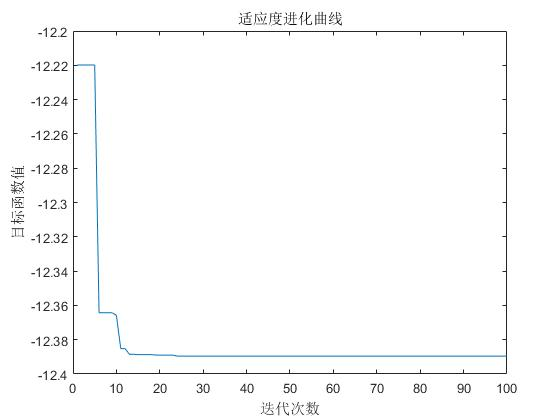展开全文遗传算法
• 主要介绍了JAVA得到数组中最大值和最小值的简单实例,需要的朋友可以参考下
• 通过PSO粒子群算法求最大最小值，可直接运行。粒子群算法通过设计一种无质量的粒子来模拟鸟群中的鸟，粒子仅具有两个属性：速度和位置，速度代表移动的快慢，位置代表移动的方向。每个粒子在搜索空间中单独的搜寻最...
• 本篇文章主要介绍了求js数组的最大值和最小值的四种方法，具有很好的参考价值。下面跟着小编一起来看下吧
• 利用粒子群算法优化方程最小值，方程变量个数可变，方程可自行定义，运行结果正确。粒子群算法（ Particle Swarm Optimization, PSO）最早是由Eberhart和Kennedy于1995年提出，它的基本概念源于对鸟群觅食行为的研究...
• 1、ArcTools——Data Management Tools ——Raster——Raster Properties

1、ArcTools——Data Management Tools ——Raster——Raster Properties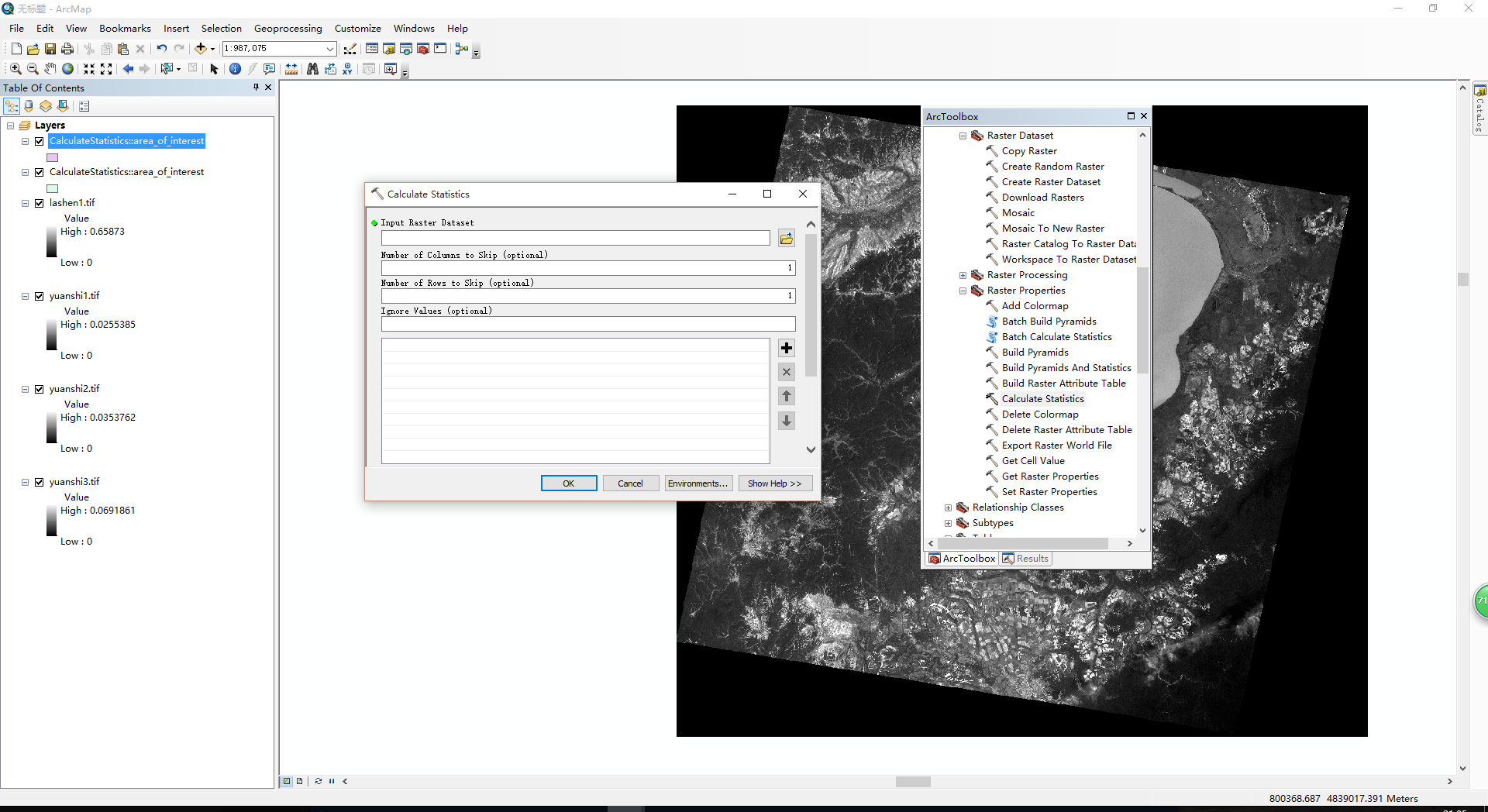展开全文ArcGIS
• java利用数组求平均值,最大值,最小值。需要的朋友可以过来参考下，希望对大家有所帮助
• C语言程序设计-给定n个数据, 求最小值出现的位置（如果最小值出现多次,求出第一次出现的位置即可）;.c...